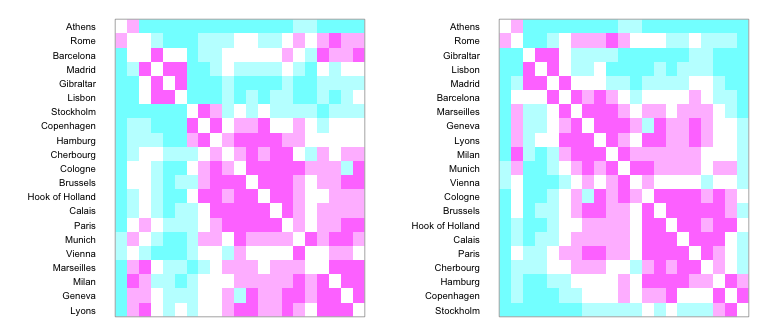# Clustering Graphics

#### 2019-01-07

This package will order panels in scatterplot matrices and parallel coordinate displays by some merit index. The package contains various indices of merit, ordering functions, and enhanced versions of pairs and parcoord which color panels according to their merit level. For details on the methods used, consult “Clustering Visualisations of Multidimensional Data”, Journal of Computational and Graphical Statistics, vol. 13, (4), pp 788-806, 2004.

## Displaying a correlation matrix

library(gclus)
#> Loading required package: cluster
data(longley)
longley.cor <- cor(longley)
longley.color <- dmat.color(longley.cor)

dmat.color assigns three colours to the correlations according to the correlation magnitude. High correlations are in pink, the middle third are in blue, and the botom third are in yellow.

par(mar=c(1,1,1,1))
plotcolors(longley.color,dlabels=rownames(longley.color))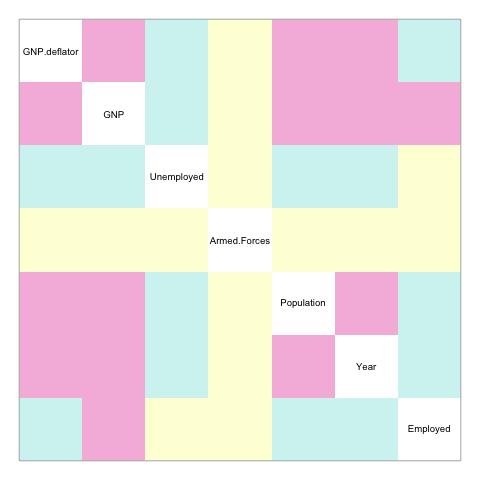If you want to change the colour scheme:

longley.color <- dmat.color(longley.cor, byrank=FALSE)
longley.color <- dmat.color(longley.cor, breaks=c(-1,0,.5,.8,1),
cm.colors(4))

The plot is easier to interpret if variables are reorded prior to plotting.

par(mar=c(1,1,1,1))
longley.o <- order.hclust(longley.cor)
longley.color1 <- longley.color[longley.o,longley.o]
plotcolors(longley.color1,dlabels=rownames(longley.color1))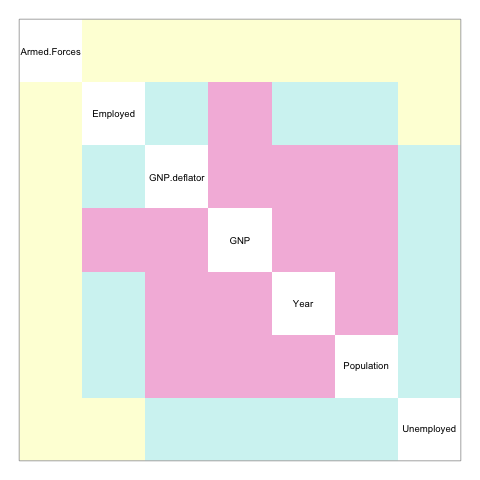## Displaying a pairs plot with coloured panels

cpairs is a version of pairs All the high-correlation panels appear together in a block.

par(mar=c(1,1,1,1))
cpairs(longley, order= longley.o,panel.color= longley.color)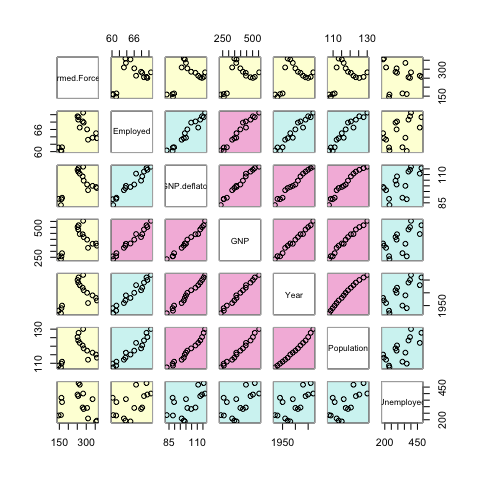If the order is not supplied, then the variables are plotted in default dataset order.

## Displaying a PCP plot with coloured panels

cparcoord is a versions of parcoord where panels can be coloured. Again, the pink panels have high correlation, blue panels have middling correlation, and yellow panels have low correlation.

cparcoord(longley, order= longley.o,panel.color= longley.color,
horizontal=TRUE, mar=c(2,4,1,1))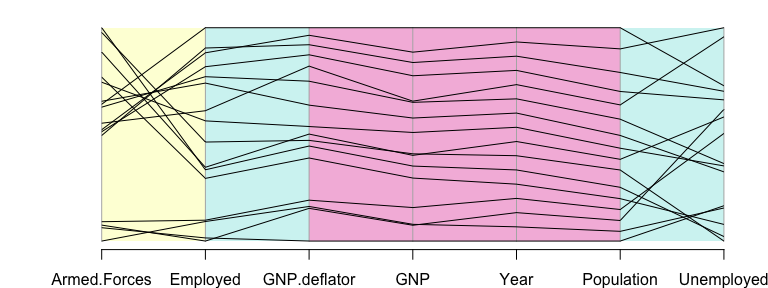## Plotting re-ordered dendrograms.

eurodist is a built-in distance matrix giving the distance between European cities.

par(mar=c(1,1,1,1))
data(eurodist)
dis <- as.dist(eurodist)
hc <- hclust(dis, "ave")
plot(hc)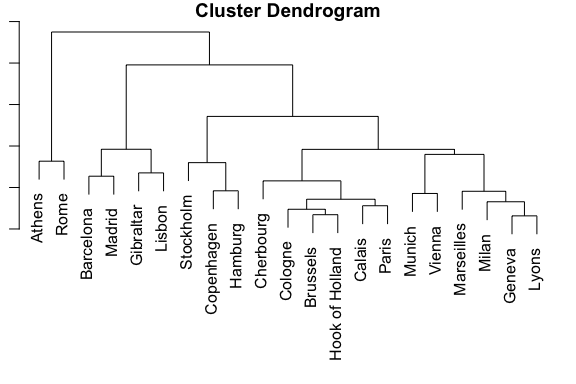order.hclust re-orders a dendrogram to improve the similarity between nearby leaves. Applying it to the hc object:

par(mar=c(1,1,1,1))
hc1 <- reorder.hclust(hc, dis)
plot(hc1)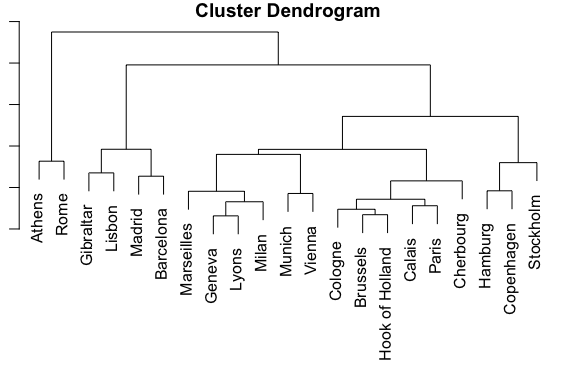Both dendrograms correspond to the same tree structure, but the second one shows that Paris is closer to Cherbourg than Munich, and Rome is closer to Gibralter than to Barcelona.

We can also compare both orderings with an image plot of the colors. The second ordering seems to place nearby cities closer to each other.


layout(matrix(1:2,nrow=1,ncol=2))
par(mar=c(1,6,1,1))
cmat <- dmat.color(eurodist, rev(cm.colors(5)))
plotcolors(cmat[hc$order,hc$order], rlabels=labels(eurodist)[hc$order]) plotcolors(cmat[hc1$order,hc1$order], rlabels=labels(eurodist)[hc1$order])`